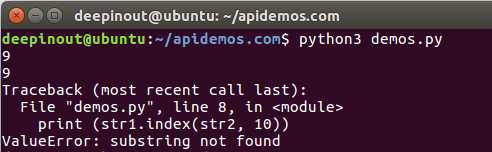# Python String index()方法

## Python String index()方法

index() 方法检测字符串中是否包含子字符串 str ，如果指定 beg（开始） 和 end（结束） 范围，则检查是否包含在指定范围内，该方法与 python find()方法一样，只不过如果str不在 string中会报一个异常。

## Python String index() 语法

index()方法语法：

``str.index(str, beg=0, end=len(string))``

## Python String index() 参数

• str - 指定检索的字符串
• beg - 开始索引，默认为0。
• end - 结束索引，默认为字符串的长度。

## Python String index() 示例1

``````#!/usr/bin/python3

str1 = "apidemos example....wow!!!"
str2 = "exam";

print (str1.index(str2))       # Basic usage of Python String index() Method
print (str1.index(str2, 5))    # Python String index() With start and end Arguments
print (str1.index(str2, 10))   # Exception when using Python String index() Method``````• 微信订阅
• 回顶